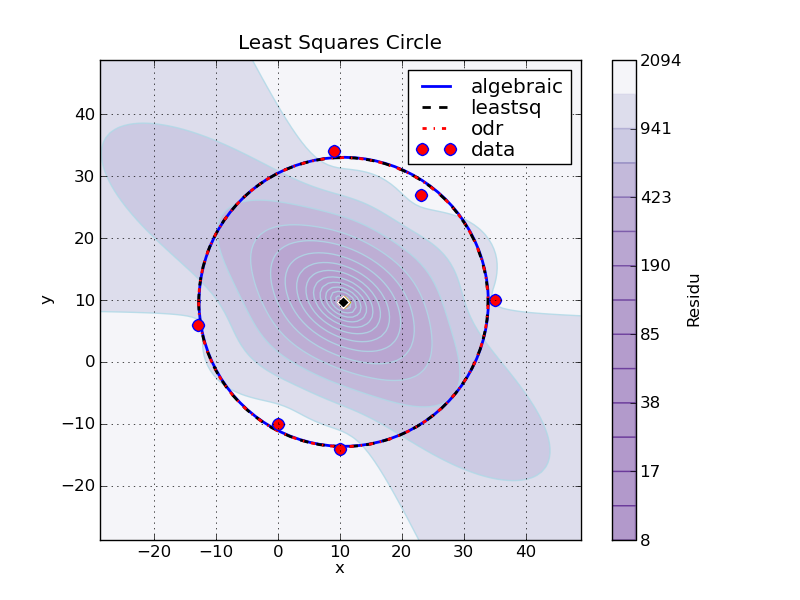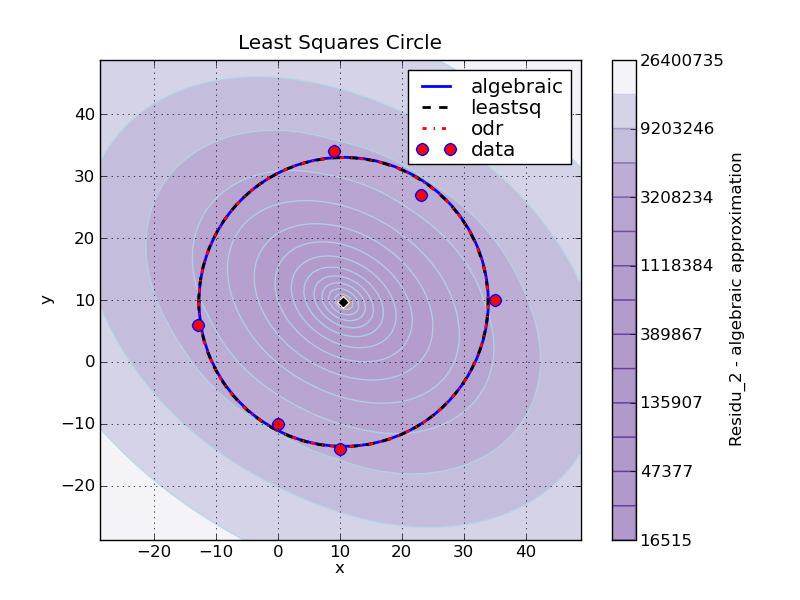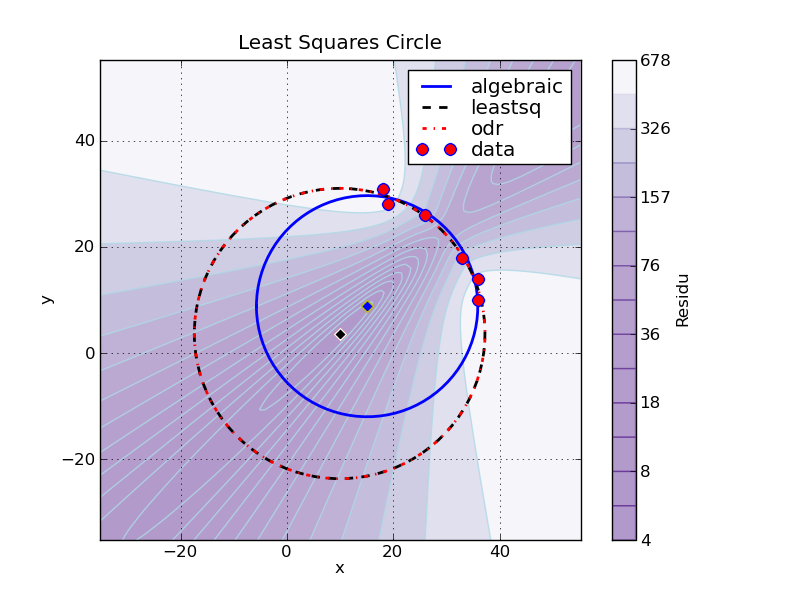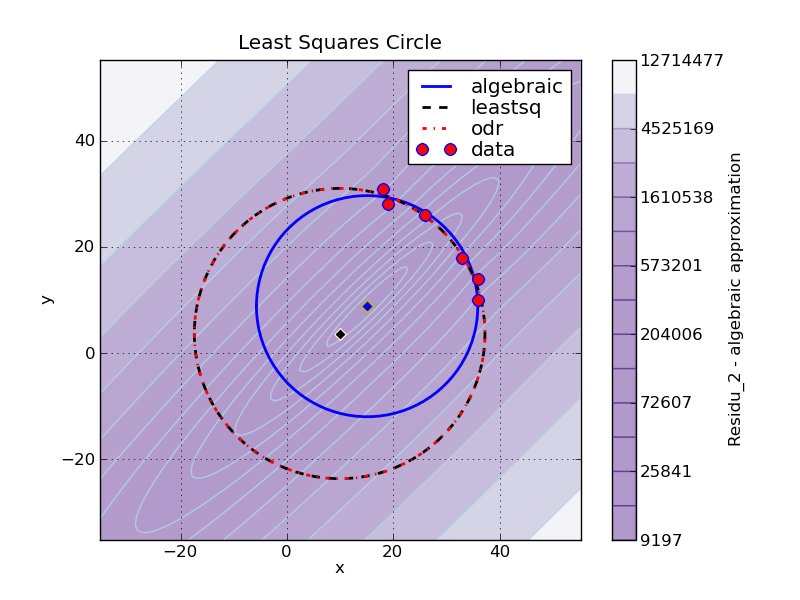This is an archival dump of old wiki content --- see scipy.org for current material.
Please see http://scipy-cookbook.readthedocs.org/

## Introduction

This page gathers different methods used to find the least squares circle fitting a set of 2D points (x,y).

The full code of this analysis is available here: least_squares_circle_v1d.py.

Finding the least squares circle corresponds to finding the center of the circle (xc, yc) and its radius Rc which minimize the residu function defined below:

```   1 Ri = sqrt( (x - xc)**2 + (y - yc)**2)
2 residu = sum( (Ri - Rc)**2)
```

This is a nonlinear problem. We well see three approaches to the problem, and compare there results, as well as their speeds.

## Using an algebraic approximation

As detailed in this document this problem can be approximated by a linear one if we define the function to minimize as follow:

```   1 residu_2 = sum( (Ri**2 - Rc**2)**2)
```

This leads to the following method, using linalg.solve :

```   1 # == METHOD 1 ==
2 method_1 = 'algebraic'
3
4 # coordinates of the barycenter
5 x_m = mean(x)
6 y_m = mean(y)
7
8 # calculation of the reduced coordinates
9 u = x - x_m
10 v = y - y_m
11
12 # linear system defining the center (uc, vc) in reduced coordinates:
13 #    Suu * uc +  Suv * vc = (Suuu + Suvv)/2
14 #    Suv * uc +  Svv * vc = (Suuv + Svvv)/2
15 Suv  = sum(u*v)
16 Suu  = sum(u**2)
17 Svv  = sum(v**2)
18 Suuv = sum(u**2 * v)
19 Suvv = sum(u * v**2)
20 Suuu = sum(u**3)
21 Svvv = sum(v**3)
22
23 # Solving the linear system
24 A = array([ [ Suu, Suv ], [Suv, Svv]])
25 B = array([ Suuu + Suvv, Svvv + Suuv ])/2.0
26 uc, vc = linalg.solve(A, B)
27
28 xc_1 = x_m + uc
29 yc_1 = y_m + vc
30
31 # Calcul des distances au centre (xc_1, yc_1)
32 Ri_1     = sqrt((x-xc_1)**2 + (y-yc_1)**2)
33 R_1      = mean(Ri_1)
34 residu_1 = sum((Ri_1-R_1)**2)
```

## Using scipy.optimize.leastsq

Scipy comes will several tools to solve the nonlinear problem above. Among them, scipy.optimize.leastsq is very simple to use in this case.

Indeed, once the center of the circle is defined, the radius can be calculated directly and is equal to mean(Ri). So there is only two parameters left: xc and yc.

### Basic usage

```   1 #  == METHOD 2 ==
2 from scipy      import optimize
3
4 method_2 = "leastsq"
5
6 def calc_R(xc, yc):
7     """ calculate the distance of each 2D points from the center (xc, yc) """
8     return sqrt((x-xc)**2 + (y-yc)**2)
9
10 def f_2(c):
11     """ calculate the algebraic distance between the data points and the mean circle centered at c=(xc, yc) """
12     Ri = calc_R(*c)
13     return Ri - Ri.mean()
14
15 center_estimate = x_m, y_m
16 center_2, ier = optimize.leastsq(f_2, center_estimate)
17
18 xc_2, yc_2 = center_2
19 Ri_2       = calc_R(*center_2)
20 R_2        = Ri_2.mean()
21 residu_2   = sum((Ri_2 - R_2)**2)
```

### Advanced usage, with jacobian function

To gain in speed, it is possible to tell optimize.leastsq how to compute the jacobian of the function by adding a Dfun argument:

```   1 # == METHOD 2b ==
2 method_2b  = "leastsq with jacobian"
3
4 def calc_R(xc, yc):
5     """ calculate the distance of each data points from the center (xc, yc) """
6     return sqrt((x-xc)**2 + (y-yc)**2)
7
8 def f_2b(c):
9     """ calculate the algebraic distance between the 2D points and the mean circle centered at c=(xc, yc) """
10     Ri = calc_R(*c)
11     return Ri - Ri.mean()
12
13 def Df_2b(c):
14     """ Jacobian of f_2b
15     The axis corresponding to derivatives must be coherent with the col_deriv option of leastsq"""
16     xc, yc     = c
17     df2b_dc    = empty((len(c), x.size))
18
19     Ri = calc_R(xc, yc)
20     df2b_dc = (xc - x)/Ri                   # dR/dxc
21     df2b_dc = (yc - y)/Ri                   # dR/dyc
22     df2b_dc    = df2b_dc - df2b_dc.mean(axis=1)[:, newaxis]
23
24     return df2b_dc
25
26 center_estimate = x_m, y_m
27 center_2b, ier = optimize.leastsq(f_2b, center_estimate, Dfun=Df_2b, col_deriv=True)
28
29 xc_2b, yc_2b = center_2b
30 Ri_2b        = calc_R(*center_2b)
31 R_2b         = Ri_2b.mean()
32 residu_2b    = sum((Ri_2b - R_2b)**2)
```

## Using scipy.odr

Scipy has a dedicated package to deal with orthogonal distance regression, namely scipy.odr. This package can handle both explict and implicit function definition, and we will used the second form in this case.

Here is the implicit definition of the circle:

```   1 (x - xc)**2 + (y-yc)**2 - Rc**2 = 0
```

### Basic usage

This leads to the following code:

```   1 # == METHOD 3 ==
2 from scipy      import  odr
3
4 method_3 = "odr"
5
6 def f_3(beta, x):
7     """ implicit definition of the circle """
8     return (x-beta)**2 + (x-beta)**2 -beta**2
9
10 # initial guess for parameters
11 R_m = calc_R(x_m, y_m).mean()
12 beta0 = [ x_m, y_m, R_m]
13
14 # for implicit function :
15 #       data.x contains both coordinates of the points (data.x = [x, y])
16 #       data.y is the dimensionality of the response
17 lsc_data  = odr.Data(row_stack([x, y]), y=1)
18 lsc_model = odr.Model(f_3, implicit=True)
19 lsc_odr   = odr.ODR(lsc_data, lsc_model, beta0)
20 lsc_out   = lsc_odr.run()
21
22 xc_3, yc_3, R_3 = lsc_out.beta
23 Ri_3 = calc_R([xc_3, yc_3])
24 residu_3 = sum((Ri_3 - R_3)**2)
```

### Advanced usage, with jacobian functions

One of the advantages of the implicit function definition is that its derivatives are very easily calculated.

This can be used to complete the model:

```   1 # == METHOD 3b ==
2 method_3b  = "odr with jacobian"
3
4 def f_3b(beta, x):
5     """ implicit definition of the circle """
6     return (x-beta)**2 + (x-beta)**2 -beta**2
7
8 def jacb(beta, x):
9     """ Jacobian function with respect to the parameters beta.
10     return df_3b/dbeta
11     """
12     xc, yc, r = beta
13     xi, yi    = x
14
15     df_db    = empty((beta.size, x.shape))
16     df_db =  2*(xc-xi)                     # d_f/dxc
17     df_db =  2*(yc-yi)                     # d_f/dyc
18     df_db = -2*r                           # d_f/dr
19
20     return df_db
21
22 def jacd(beta, x):
23     """ Jacobian function with respect to the input x.
24     return df_3b/dx
25     """
26     xc, yc, r = beta
27     xi, yi    = x
28
29     df_dx    = empty_like(x)
30     df_dx =  2*(xi-xc)                     # d_f/dxi
31     df_dx =  2*(yi-yc)                     # d_f/dyi
32
33     return df_dx
34
35 def calc_estimate(data):
36     """ Return a first estimation on the parameter from the data  """
37     xc0, yc0 = data.x.mean(axis=1)
38     r0 = sqrt((data.x-xc0)**2 +(data.x -yc0)**2).mean()
39     return xc0, yc0, r0
40
41 # for implicit function :
42 #       data.x contains both coordinates of the points
43 #       data.y is the dimensionality of the response
44 lsc_data  = odr.Data(row_stack([x, y]), y=1)
45 lsc_model = odr.Model(f_3b, implicit=True, estimate=calc_estimate, fjacd=jacd, fjacb=jacb)
46 lsc_odr   = odr.ODR(lsc_data, lsc_model)    # beta0 has been replaced by an estimate function
47 lsc_odr.set_job(deriv=3)                    # use user derivatives function without checking
48 lsc_odr.set_iprint(iter=1, iter_step=1)     # print details for each iteration
49 lsc_out   = lsc_odr.run()
50
51 xc_3b, yc_3b, R_3b = lsc_out.beta
52 Ri_3b       = calc_R(xc_3b, yc_3b)
53 residu_3b   = sum((Ri_3b - R_3b)**2)
```

## Comparison of the three methods

We will compare the results of these three methods in two cases:

• when 2D points are all around the circle
• when 2D points are in a small arc

### Data points all around the circle

Here is an example with data points all around the circle:

```   1 # Coordinates of the 2D points
2 x = r_[  9,  35, -13,  10,  23,   0]
3 y = r_[ 34,  10,   6, -14,  27, -10]
```

This gives:

 SUMMARY Method Xc Yc Rc nb_calls std(Ri) residu residu2 algebraic 10.55231 9.70590 23.33482 1 1.135135 7.731195 16236.34 leastsq 10.50009 9.65995 23.33353 15 1.133715 7.711852 16276.89 leastsq with jacobian 10.50009 9.65995 23.33353 7 1.133715 7.711852 16276.89 odr 10.50009 9.65995 23.33353 82 1.133715 7.711852 16276.89 odr with jacobian 10.50009 9.65995 23.33353 16 1.133715 7.711852 16276.89

Note:

• nb_calls correspond to the number of function calls of the function to be minimized, and do not take into account the number of calls to derivatives function. This differs from the number of iteration as ODR can make multiple calls during an iteration.

• as shown on the figures below, the two functions residu and residu_2 are not equivalent, but their minima are close in this case.### Data points around an arc

Here is an example where data points form an arc:

```   1 x = r_[36, 36, 19, 18, 33, 26]
2 y = r_[14, 10, 28, 31, 18, 26]
```
 SUMMARY Method Xc Yc Rc nb_calls std(Ri) residu residu2 algebraic 15.05503 8.83615 20.82995 1 0.930508 5.195076 9153.40 leastsq 9.88760 3.68753 27.35040 24 0.820825 4.042522 12001.98 leastsq with jacobian 9.88759 3.68752 27.35041 10 0.820825 4.042522 12001.98 odr 9.88757 3.68750 27.35044 472 0.820825 4.042522 12002.01 odr with jacobian 9.88757 3.68750 27.35044 109 0.820825 4.042522 12002.01### Conclusion

ODR and leastsq gives the same results.

Optimize.leastsq is the most efficient method, and can be two to ten times faster than ODR, at least as regards the number of function call.

Adding a function to compute the jacobian can lead to decrease the number of function calls by a factor of two to five.

In this case, to use ODR seems a bit overkill but it can be very handy for more complex use cases like ellipses.

The algebraic approximation gives good results when the points are all around the circle but is limited when there is only an arc to fit.

Indeed, the two errors functions to minimize are not equivalent when data points are not all exactly on a circle. The algebraic method leads in most of the case to a smaller radius than that of the least squares circle, as its error function is based on squared distances and not on the distance themselves.

SciPy: Cookbook/Least_Squares_Circle (last edited 2015-10-24 17:48:23 by anonymous)